# 三、轮廓和分割

• 使用大津算法将灰度图像二值化
• 在二进制图像中查找外部和内部轮廓
• 从二进制图像中提取连通组件
• 将线和圆拟合为二维点集
• 计算图像矩
• 使用曲线 - 近似值，长度和面积
• 检查点是否在轮廓内
• 计算从每个像素到二维点集的距离
• 使用 K 均值算法的图像分割
• 使用分割种子的图像分割，分水岭算法

# 操作步骤

1. 导入模块：
import cv2
import numpy as np
import matplotlib.pyplot as plt

1. 读取测试图像：
image = cv2.imread('../data/Lena.png', 0)

1. 使用大津法估计阈值：
otsu_thr, otsu_mask = cv2.threshold(image, -1, 1, cv2.THRESH_BINARY | cv2.THRESH_OTSU)
print('Estimated threshold (Otsu):', otsu_thr)

1. 可视化结果：
plt.figure()
plt.subplot(121)
plt.axis('off')
plt.title('original')
plt.imshow(image, cmap='gray')
plt.subplot(122)
plt.axis('off')
plt.title('Otsu threshold')
plt.tight_layout()
plt.show()


# 工作原理

Estimated threshold (Otsu): 116.0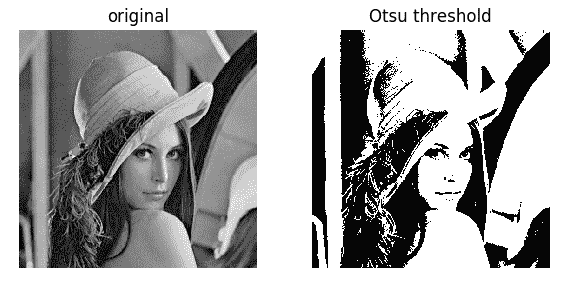# 操作步骤

1. 导入模块：
import cv2
import numpy as np
import matplotlib.pyplot as plt

1. 加载测试二进制图像：
image = cv2.imread('../data/BnW.png', 0)

1. 找到外部和内部轮廓。 将它们分为两级：
_, contours, hierarchy = cv2.findContours(image, cv2.RETR_CCOMP, cv2.CHAIN_APPROX_SIMPLE)

1. 准备外部轮廓二进制掩码：
image_external = np.zeros(image.shape, image.dtype)
for i in range(len(contours)):
if hierarchy[i] == -1:
cv2.drawContours(image_external, contours, i,
255, -1)

1. 准备内部轮廓二进制掩码：
image_internal = np.zeros(image.shape, image.dtype)
for i in range(len(contours)):
if hierarchy[i] != -1:
cv2.drawContours(image_internal, contours, i,
255, -1)

1. 可视化结果：
plt.figure(figsize=(10,3))
plt.subplot(131)
plt.axis('off')
plt.title('original')
plt.imshow(image, cmap='gray')
plt.subplot(132)
plt.axis('off')
plt.title('external')
plt.imshow(image_external, cmap='gray')
plt.subplot(133)
plt.axis('off')
plt.title('internal')
plt.imshow(image_internal, cmap='gray')
plt.tight_layout()
plt.show()


# 工作原理

• cv2.RETR_EXTERNAL：仅提取外部轮廓
• cv2.RETR_CCOMP：用于提取内部和外部轮廓，并将它们组织为两级层次结构
• cv2.RETR_TREE：用于提取内部和外部轮廓，并将它们组织成树状图
• cv2.RETR_LIST：用于在不建立任何关系的情况下提取所有轮廓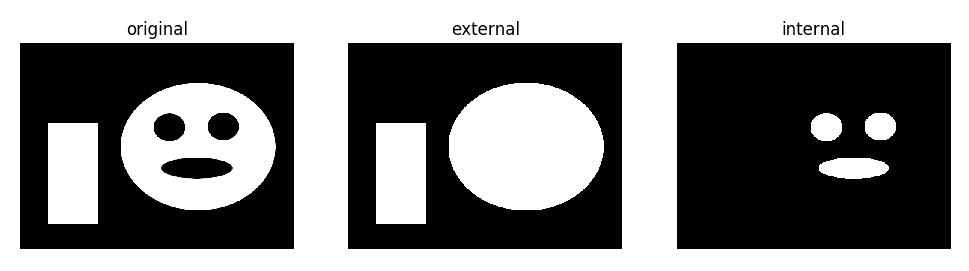# 操作步骤

1. 首先，我们导入所需的所有模块：
import cv2
import numpy as np

1. 打开图像并在其中找到连通组件：
img = cv2.imread('../data/BnW.png', cv2.IMREAD_GRAYSCALE)

connectivity = 8
num_labels, labelmap = cv2.connectedComponents(img, connectivity, cv2.CV_32S)

1. 显示原始图像和带有标签的缩放图像：
img = np.hstack((img, labelmap.astype(np.float32)/(num_labels - 1)))
cv2.imshow('Connected components', img)
cv2.waitKey()
cv2.destroyAllWindows()

1. 打开另一个图像，找到其大津遮罩，并获得连通组件及其统计信息：
img = cv2.imread('../data/Lena.png', cv2.IMREAD_GRAYSCALE)
otsu_thr, otsu_mask = cv2.threshold(img, -1, 1, cv2.THRESH_BINARY | cv2.THRESH_OTSU)


1. 过滤出面积较小的组件并创建彩色图像，在该图像上绘制具有单独颜色的其余组件以及每个组件的中心。 然后，显示结果：
num_labels, labelmap, stats, centers = output

colored = np.full((img.shape, img.shape, 3), 0, np.uint8)

for l in range(1, num_labels):
if stats[l] > 200:
colored[labelmap == l] = (0, 255*l/num_labels, 255*num_labels/l)
cv2.circle(colored,
(int(centers[l]), int(centers[l])), 5, (255, 0, 0), cv2.FILLED)

cv2.imshow('Connected components', np.hstack((img, colored)))
cv2.waitKey()
cv2.destroyAllWindows()


# 工作原理

OpenCV 中有两个函数可用于查找连通组件：cv2.connectedComponentscv2.connectedComponentsWithStats。 两者都采用相同的参数：要查找其组件的二进制图像，连接类型和输出图像的深度，以及组件的标签。 返回值会有所不同。

cv2.connectedComponents更简单，它返回一个组件编号的元组和一个带有组件标签的图像（labelmap）。 除了前一个函数的输出外，cv2.connectedComponentsWithStats还返回有关每个组件及其组件质心位置的统计信息。# 操作步骤

1. 首先，导入所有模块：
import cv2
import numpy as np
import random

1. 在要绘制的图像上创建图像，并随机生成椭圆的参数，例如半轴长度和旋转角度：
img = np.full((512, 512, 3), 255, np.uint8)

axes = (int(256*random.uniform(0, 1)), int(256*random.uniform(0, 1)))
angle = int(180*random.uniform(0, 1))
center = (256, 256)

1. 使用找到的参数为椭圆生成点，并向它们添加随机噪声：
pts = cv2.ellipse2Poly(center, axes, angle, 0, 360, 1)
pts += np.random.uniform(-10, 10, pts.shape).astype(np.int32)

1. 在图像上绘制椭圆和生成的点，然后显示图像：
cv2.ellipse(img, center, axes, angle, 0, 360, (0, 255, 0), 3)

for pt in pts:
cv2.circle(img, (int(pt), int(pt)), 3, (0, 0, 255))

cv2.imshow('Fit ellipse', img)
cv2.waitKey()
cv2.destroyAllWindows()

1. 找到最适合我们的噪点的椭圆参数，在图像上绘制结果椭圆，然后显示它：
ellipse = cv2.fitEllipse(pts)
cv2.ellipse(img, ellipse, (0, 0, 0), 3)

cv2.imshow('Fit ellipse', img)
cv2.waitKey()
cv2.destroyAllWindows()

1. 创建清晰的图像，为y = x函数生成点，并向它们添加随机噪声：
img = np.full((512, 512, 3), 255, np.uint8)

pts = np.arange(512).reshape(-1, 1)
pts = np.hstack((pts, pts))
pts += np.random.uniform(-10, 10, pts.shape).astype(np.int32)

1. 绘制y = x函数并生成点； 然后，显示图像：
cv2.line(img, (0,0), (512, 512), (0, 255, 0), 3)

for pt in pts:
cv2.circle(img, (int(pt), int(pt)), 3, (0, 0, 255))

cv2.imshow('Fit line', img)
cv2.waitKey()
cv2.destroyAllWindows()

1. 找到噪声点的直线参数，绘制结果并显示图像：
vx,vy,x,y = cv2.fitLine(pts, cv2.DIST_L2, 0, 0.01, 0.01)
y0 = int(y - x*vy/vx)
y1 = int((512 - x)*vy/vx + y)
cv2.line(img, (0, y0), (512, y1), (0, 0, 0), 3)

cv2.imshow('Fit line', img)
cv2.waitKey()
cv2.destroyAllWindows()


# 工作原理

cv2.fitEllipse仅接受一组二维点的参数，我们需要为其找到曲线参数，然后返回找到的参数，中心点，半轴长度和旋转角度。 当我们要显示结果时，这些参数可以直接传递到cv2.ellipse绘图函数。

# 操作步骤

1. 导入模块：
import cv2
import numpy as np
import matplotlib.pyplot as plt

1. 在黑色背景上绘制一个测试图像-以点（320240）为中心的白色椭圆：
image = np.zeros((480, 640), np.uint8)
cv2.ellipse(image, (320, 240), (200, 100), 0, 0, 360, 255, -1)

1. 计算矩并打印其值：
m = cv2.moments(image)
for name, val in m.items():
print(name, '\t', val)

1. 执行一个简单的测试，以检查计算出的矩是否有意义，并使用图像的第一个矩来计算图像质心。 它必须靠近我们在上面指定的椭圆的中心：
print('Center X estimated:', m['m10'] / m['m00'])
print('Center Y estimated:', m['m01'] / m['m00'])


# 工作原理

nu11     -2.809466679966455e-13
mu12     -422443285.20703125
mu21     -420182048.71875
m11      1237939564800.0
mu20     161575917357.31616
m10      5158101240.0
nu03     1.013174855849065e-10
nu12     -4.049505150683136e-10
nu21     -4.0278291313762605e-10
mu03     105694127.71875
nu30     1.618061841335058e-09
m30      683285449618080.0
nu02     0.00015660970937729079
m20      1812142855350.0
m00      16119315.0
mu02     40692263506.42969
nu20     0.0006218468887998859
m02      969157708320.0
m21      434912202354750.0
m01      3868620810.0
m03      252129278267070.0
mu11     -72.9990234375
mu30     1687957749.125
m12      310125260718570.0


Center X estimated: 319.9950643063927
Center Y estimated: 239.999082467214


# 操作步骤

1. 导入所有必需的模块，打开一个图像，然后在屏幕上显示它：
import cv2, random
import numpy as np

1. 找到加载的图像的轮廓，绘制它们，然后显示结果：
im2, contours, hierarchy = cv2.findContours(img, cv2.RETR_TREE, cv2.CHAIN_APPROX_SIMPLE)

color = cv2.cvtColor(img, cv2.COLOR_GRAY2BGR)
cv2.drawContours(color, contours, -1, (0,255,0), 3)

cv2.imshow('contours', color)
cv2.waitKey()
cv2.destroyAllWindows()

1. 取第一个轮廓，在各种情况下找到其面积，并输出结果数字：
contour = contours

print('Area of contour is %.2f' % cv2.contourArea(contour))
print('Signed area of contour is %.2f' % cv2.contourArea(contour, True))
print('Signed area of contour is %.2f' % cv2.contourArea(contour[::-1], True))

1. 找到轮廓的长度，然后打印：
print('Length of closed contour is %.2f' % cv2.arcLength(contour, True))
print('Length of open contour is %.2f' % cv2.arcLength(contour, False))

1. 找到轮廓的凸包，在图像上绘制并显示：
hull = cv2.convexHull(contour)
cv2.drawContours(color, [hull], -1, (0,0,255), 3)

cv2.imshow('contours', color)
cv2.waitKey()
cv2.destroyAllWindows()

1. 检查轮廓及其外壳的凸度：
print('Convex status of contour is %s' % cv2.isContourConvex(contour))
print('Convex status of its hull is %s' % cv2.isContourConvex(hull))

1. 创建一个带有轨迹栏的窗口，以控制轮廓近似的质量，找到轮廓近似并显示结果：
cv2.namedWindow('contours')

img = np.copy(color)

def trackbar_callback(value):
global img
epsilon = value*cv2.arcLength(contour, True)*0.1/255
approx = cv2.approxPolyDP(contour, epsilon, True)
img = np.copy(color)
cv2.drawContours(img, [approx], -1, (255,0,255), 3)

cv2.createTrackbar('Epsilon', 'contours', 1, 255, lambda v: trackbar_callback(v))
while True:
cv2.imshow('contours', img)
key = cv2.waitKey(3)
if key == 27:
break

cv2.destroyAllWindows()


# 工作原理

cv2.contourArea计算轮廓的面积，顾名思义。 它以一个表示轮廓的点集作为其第一个参数，并使用一个布尔标志作为其第二个参数。 该例程返回轮廓的浮点面积。 该标志允许我们计算有符号（当True时）或无符号（当False时）区域，其中符号代表轮廓中点的顺时针或逆时针顺序。 关于cv2.contourArea的重要说明是，不能保证该区域对于具有自相交的轮廓是正确的。

cv2.convexHull可帮助您找到轮廓的凸包。 它以轮廓为参数，并返回其凸包（也是轮廓）。 另外，您可以使用cv2.isContourConvex函数检查轮廓的凸度，只需将轮廓作为参数传递，当传递的轮廓为凸形时，返回值为True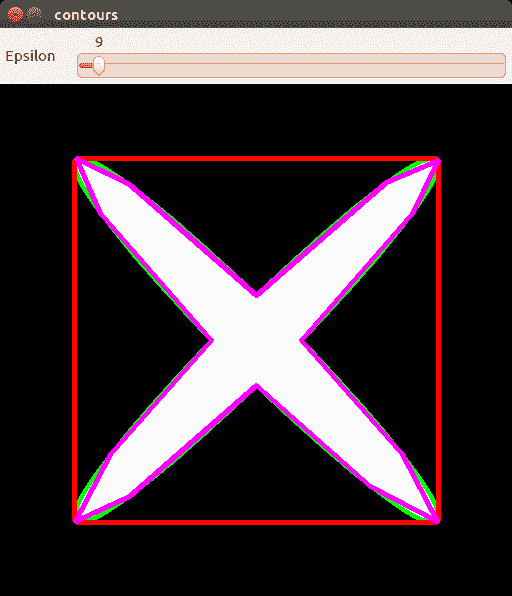# 操作步骤

1. 导入所有必需的模块，打开一个图像，然后在屏幕上显示它：
import cv2, random
import numpy as np

1. 找到图像的轮廓并显示它们：
im2, contours, hierarchy = cv2.findContours(img, cv2.RETR_TREE, cv2.CHAIN_APPROX_SIMPLE)

color = cv2.cvtColor(img, cv2.COLOR_GRAY2BGR)
cv2.drawContours(color, contours, -1, (0,255,0), 3)

cv2.imshow('contours', color)
cv2.waitKey()
cv2.destroyAllWindows()

1. 定义一个回调函数来处理用户单击图像。 此函数在发生点击的地方绘制一个小圆圈，圆圈的颜色取决于点击是在轮廓内部还是外部：
contour = contours
image_to_show = np.copy(color)
measure = True

def mouse_callback(event, x, y, flags, param):
global contour, image_to_show

if event == cv2.EVENT_LBUTTONUP:
distance = cv2.pointPolygonTest(contour, (x,y), measure)
image_to_show = np.copy(color)
if distance > 0:
pt_color = (0, 255, 0)
elif distance < 0:
pt_color = (0, 0, 255)
else:
pt_color = (128, 0, 128)
cv2.circle(image_to_show, (x,y), 5, pt_color, -1)
cv2.putText(image_to_show, '%.2f' % distance, (0, image_to_show.shape - 5),
cv2.FONT_HERSHEY_SIMPLEX, 1, (255, 255, 255))

1. 使用我们的鼠标单击处理器显示图像。 另外，让我们跟踪M按钮的按下，以切换由于cv2.pointPolygonTest函数而得到的结果的模式：
cv2.namedWindow('contours')
cv2.setMouseCallback('contours', mouse_callback)

while(True):
cv2.imshow('contours', image_to_show)
k = cv2.waitKey(1)

if k == ord('m'):
measure = not measure
elif k == 27:
break

cv2.destroyAllWindows()


# 工作原理

OpenCV 中有一个特殊函数，可测量从点到轮廓的最小距离。 称为cv2.pointPolygonTest。 它接受三个参数，并返回测得的距离。 参数是轮廓，点和布尔标志，我们将在稍后讨论它们的目的。 结果距离可以为正，负或等于零，分别对应于轮廓内部，轮廓外部或轮廓点位置。 最后一个布尔参数确定我们的函数返回的是精确距离还是仅返回具有值的指示符（+1; 0; -1）。 指示器的符号与计算精确距离的模式具有相同的含义。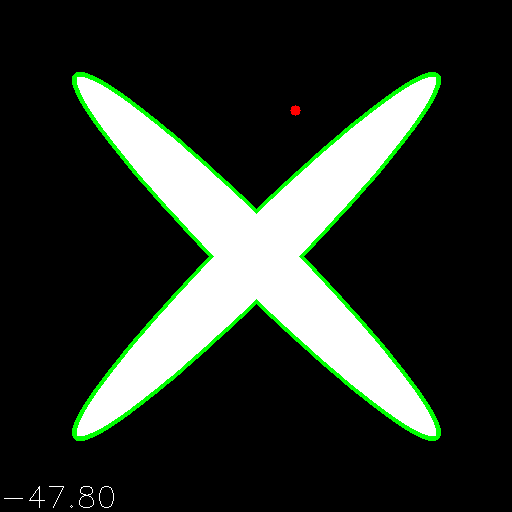# 操作步骤

1. 导入模块：
import cv2
import numpy as np
import matplotlib.pyplot as plt

1. 在白色背景上绘制测试图像-黑色圆圈（无填充）：
image = np.full((480, 640), 255, np.uint8)
cv2.circle(image, (320, 240), 100, 0)

1. 计算从每个点到圆的距离：
distmap = cv2.distanceTransform(image, cv2.DIST_L2, cv2.DIST_MASK_PRECISE)

1. 可视化结果：
plt.figure()
plt.imshow(distmap, cmap='gray')
plt.show()


# 工作原理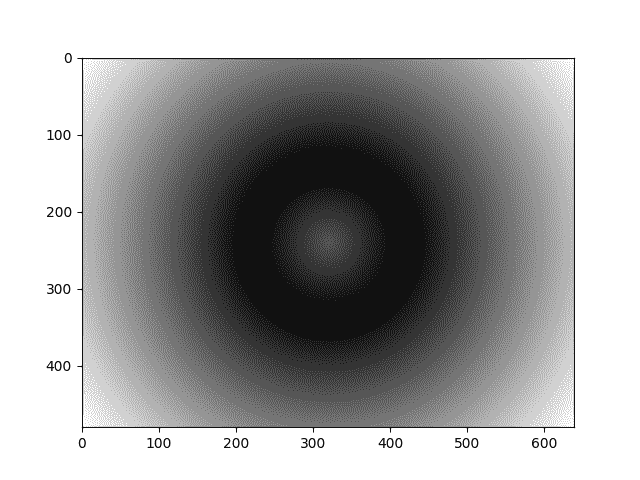# 操作步骤

1. 导入必要的模块：
import cv2
import numpy as np
import matplotlib.pyplot as plt

1. 打开图像并将其转换为 Lab 颜色空间：
image = cv2.imread('../data/Lena.png').astype(np.float32) / 255.
image_lab = cv2.cvtColor(image, cv2.COLOR_BGR2Lab)

1. 将图像重塑为向量：
data = image_lab.reshape((-1, 3))

1. 定义聚类数和完成分割过程的条件。 然后，执行 K 均值聚类：
num_classes = 4
criteria = (cv2.TERM_CRITERIA_EPS + cv2.TERM_CRITERIA_MAX_ITER, 50, 0.1)
_, labels, centers = cv2.kmeans(data, num_classes, None, criteria, 10, cv2.KMEANS_RANDOM_CENTERS)

1. 将质心的颜色应用于与这些质心相关的所有像素。 然后，将分割后的图像重新成形为原始形状。 然后，将其转换为 RGB 颜色空间：
segmented_lab = centers[labels.flatten()].reshape(image.shape)
segmented = cv2.cvtColor(segmented_lab, cv2.COLOR_Lab2RGB)

1. 一起显示原始图像和分段图像：
plt.subplot(121)
plt.axis('off')
plt.title('original')
plt.imshow(image[:, :, [2, 1, 0]])
plt.subplot(122)
plt.axis('off')
plt.title('segmented')
plt.imshow(segmented)
plt.show()


# 工作原理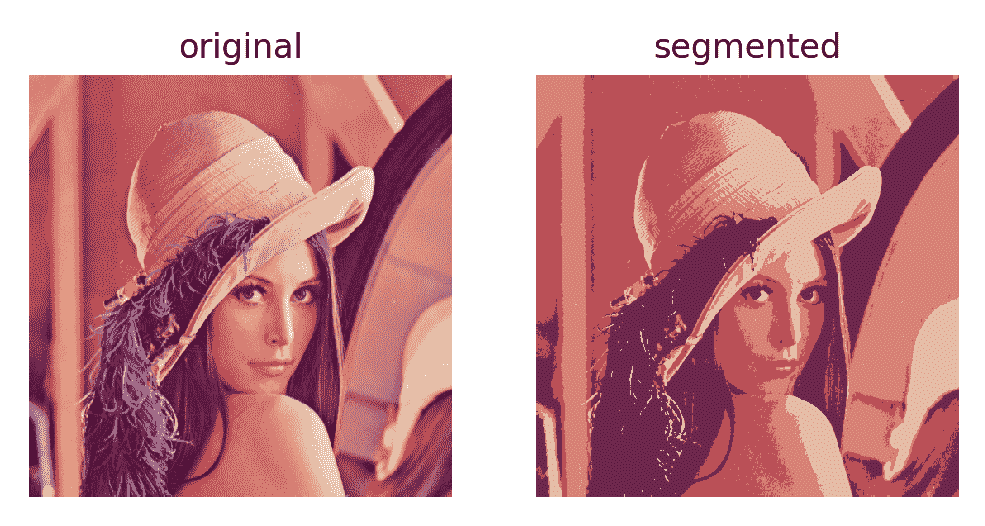# 操作步骤

1. 导入必要的模块和函数：
import cv2, random
import numpy as np
from random import randint

1. 加载图像以进行分段并创建其副本，并创建其他图像以存储种子和分段结果：
img = cv2.imread('../data/Lena.png')
show_img = np.copy(img)

seeds = np.full(img.shape[0:2], 0, np.int32)
segmentation = np.full(img.shape, 0, np.uint8)

1. 定义种子类型的数量，每种种子类型的颜色以及一些与鼠标事件一起使用的变量：
n_seeds = 9

colors = []
for m in range(n_seeds):
colors.append((255 * m / n_seeds, randint(0, 255), randint(0, 255)))

mouse_pressed = False
current_seed = 1
seeds_updated = False

1. 实现鼠标回调函数以处理鼠标事件； 让我们通过按下按钮拖动鼠标在图像上绘制种子：
def mouse_callback(event, x, y, flags, param):
global mouse_pressed, seeds_updated

if event == cv2.EVENT_LBUTTONDOWN:
mouse_pressed = True
cv2.circle(seeds, (x, y), 5, (current_seed), cv2.FILLED)
cv2.circle(show_img, (x, y), 5, colors[current_seed - 1],
cv2.FILLED)
seeds_updated = True

elif event == cv2.EVENT_MOUSEMOVE:
if mouse_pressed:
cv2.circle(seeds, (x, y), 5, (current_seed), cv2.FILLED)
cv2.circle(show_img, (x, y), 5, colors[current_seed -
1], cv2.FILLED)
seeds_updated = True

elif event == cv2.EVENT_LBUTTONUP:
mouse_pressed = False

1. 创建所有必要的窗口，设置回调，显示图像，并跟踪循环中按下的键盘按钮。 让我们通过按数字来更改当前种子以进行绘制。 并且，在完成种子更改过程后，使用分水岭算法对图像进行分割：
cv2.namedWindow('image')
cv2.setMouseCallback('image', mouse_callback)

while True:
cv2.imshow('segmentation', segmentation)
cv2.imshow('image', show_img)

k = cv2.waitKey(1)

if k == 27:
break
elif k == ord('c'):
show_img = np.copy(img)
seeds = np.full(img.shape[0:2], 0, np.int32)
segmentation = np.full(img.shape, 0, np.uint8)
elif k > 0 and chr(k).isdigit():
n = int(chr(k))
if 1 <= n <= n_seeds and not mouse_pressed:
current_seed = n

if seeds_updated and not mouse_pressed:
seeds_copy = np.copy(seeds)
cv2.watershed(img, seeds_copy)
segmentation = np.full(img.shape, 0, np.uint8)
for m in range(n_seeds):
segmentation[seeds_copy == (m + 1)] = colors[m]

seeds_updated = False

cv2.destroyAllWindows()


# 工作原理

cv2.watershed函数实现该算法，并接受两个参数，即要分割的图像和初始种子。 分割的图像应为彩色和 8 位。 种子应以与分割后的图像相同的空间大小存储在图像中，但只有一个通道和不同的深度int32。 第二个参数中应使用不同的数字表示不同的种子，其他像素应设置为零。 该例程用相关的邻近种子填充种子图像中的零值。